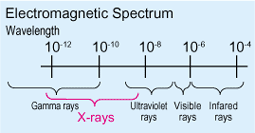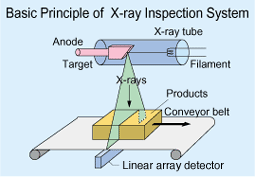# Glossary

### X-rays：

X-rays are a form of ionizing radiation. X-rays used in the inspection system are generated by an X-ray tube and are in the extremely short wavelength band of the electromagnetic spectrum (10-8 to 10-12 m or 100 to 0.01 A).### Tube current：

The tube current is the current flowing from the cathode to the anode in the X-ray tube. The amount of X-rays generated by the tube changes according to the tube current. The tube current is adjusted according to the contaminant to be detected, the type of product and the conveyor speed.

### Tube voltage：

The tube voltage is the voltage impressed between the anode and cathode. The wavelength of the X-rays becomes shorter at higher tube voltages and shorter wavelengths have higher penetration power. The tube voltage is adjusted according to the contaminant to be detected, the type of product and the conveyor speed.

### Gray absorbance (Gy)：

This is a measure of the amount of energy absorbed by the material. When 1 kg of material absorbs 1 joule (J) of energy, the amount of absorbed energy is 1 Gray (Gy).

### Sievert dose equivalent (Sv)：

The dose equivalent (Sv) expresses the impact of ionizing radiation on living tissues. It is calculated using various indices such as the Quality Factor (Q) as follows: Dose equivalent H (Sv) = D (Gy) x Q (Quality Factor) x N (Correction Factor). In the case of X-rays, both of the values of Q and N are 1, so 1 Sv = 1 Gy.